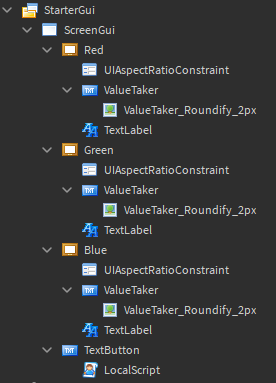# Issue transforming string to integer

Hello! This is simple, so I’ll get straight to the point.
I wanted to create 3 textboxes, which allow players to input an RGB value to change the colour of some items. This is my current script.

``````script.Parent.MouseButton1Down:Connect(function()
local Red = script.Parent.Parent.Red.ValueTaker.Text
local Green = script.Parent.Parent.Green.ValueTaker.Text
local Blue = script.Parent.Parent.Blue.ValueTaker.Text
if tonumber(Red) and tonumber(Green) and tonumber(Blue) then
local R = tonumber(Red)
local G = tonumber(Green)
local B = tonumber(Blue)
if 0 >= R <= 255 and 0 >= G <= 255 and 0 >= B <= 255 then
print(R,G,B)
end
end
end)
``````

Upon running, this error persists.
` 10:19:11.810 - Players.Loggkat.PlayerGui.ScreenGui.TextButton.LocalScript:9: attempt to compare boolean and number`

Not sure if it would help, but here is the hierarchy of the GUI, as the problem seems to be based on the logic of transforming the string to a number.Probably some small stupid mistake I’m doing, but I just can’t see what I’m doing wrong.

This should fix it:

``````if 0 >= R and R <= 255 and 0 >= G and G <= 255 and 0 >= B and B <= 255 then
print(R,G,B)
end
``````

If statements in Lua don’t work with compound inequalities. What it does in your code is get a bool from one part of the comparison and it’s comparing a bool (if the comparison is true or false) to a number, which errors.

3 Likes

Can I recommend that you just clamp the values instead?

``````local Red, Green, Blue = -50, 50, 285

R, G, B = tonumber(Red) or 0, tonumber(Green) or 0, tonumber(Blue) or 0
for i, v in next, {R = R, G = G, B = B} do
getfenv(0)[i] = math.clamp(v, 0, 255)
end

table.foreach({R, G, B}, print) --> Prints 0, 50, 255
``````

``````script.Parent.MouseButton1Down:Connect(function()
local Red = script.Parent.Parent.Red.ValueTaker.Text
local Green = script.Parent.Parent.Green.ValueTaker.Text
local Blue = script.Parent.Parent.Blue.ValueTaker.Text
if tonumber(Red) and tonumber(Green) and tonumber(Blue) then
local R = math.clamp(tonumber(Red), 0, 255)
local G = math.clamp(tonumber(Green), 0, 255)
local B = math.clamp(tonumber(Blue), 0, 255)

print(R, G, B)
end
end)
``````